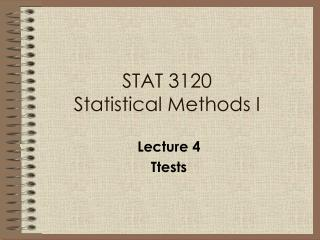# STAT 3120 Statistical Methods I - PowerPoint PPT PresentationDownload PresentationSTAT 3120 Statistical Methods I

STAT 3120 Statistical Methods IDownload Presentation## STAT 3120 Statistical Methods I

- - - - - - - - - - - - - - - - - - - - - - - - - - - E N D - - - - - - - - - - - - - - - - - - - - - - - - - - -
##### Presentation Transcript

1. STAT 3120Statistical Methods I Lecture 4 Ttests

2. STAT3120 - Ttests The term “Ttest” comes from the application of the t-distribution to evaluate a hypothesis. The t-distribution is used when the sample size is too small (less than 30) to use s/SQRT(n) as a substitute for the population std. Refer to pages 228/229 in your book for a detailed explanation of the t-distribution. In practice, even hypothesis tests with sample sizes greater than 30, which utilize the normal distribution, are commonly referred to as “ttests”. Note: a “t-statistic” and a “z-score” are conceptually similar

3. STAT3120 - Ttests The t-table (see Appendix 2) is effectively the inverse of the z-score table – the “inside” of the table includes the t-statistics while the “outside” of the table (the designation of the rows and columns) includes the degrees of freedom and the alpha values. To determine an appropriate t-statistic for a test, you must know the alpha value and the degrees of freedom (n-1). Note that as the number of observation increase, the t-distribution is assumed to approach normality.

4. STAT3120 - Ttests • Ttests take three forms: • One Sample Ttest - compares the mean of the sample to a given number. • e.g. Is average monthly revenue per customer who switches >\$50 ? • Formal Hypothesis Statement examples: • H0:   \$50 • H1:  > \$50 H0:  = \$50 H1:   \$50

5. STAT3120 - Ttests Example from page 232: After a massive outbreak of salmonella, the CDC determined that the source was from a particular manufacturer of ice cream. The CDC sampled 9 production runs if the manufacturer, with the following results (all in MPN/g): .593 .142 .329 .691 .231 .793 .519 .392 .418 Use this data to determine if the avg level of salmonella is greater than .3 MPN/g, which is considered to be dangerous.

6. STAT3120 - Ttests First, Identify the Hypothesis Statements, including the Type I and Type II errors…and your assignment of alpha. Then, do the computation by hand…

7. STAT3120 - Ttests Here is the SAS code that you need to execute a one sample ttest: Proc ttest data=<dataname> H0= <the null statement> alpha=<alpha value>; Var <quant var> ; Run;

8. STAT3120 - Ttests • Two Sample Ttest - compares the mean of the first sample minus the mean of the second sample to a given number. • e.g. Is there a difference in the production output of two facilities? • Formal Hypothesis Statement examples: • H0: a - b =0 • H1: a - b  0

9. STAT3120 - Ttests • When dealing with two sample or paired ttests, it is important to check the following assumptions: • The samples are independent • The samples have approximately equal variance • The distribution of each sample is approximately normal • Note – if the assumptions are violated and/or if the sample sizes are very small, we first try a transformation (e.g., take the log or the square root). If this does not work, then we engage in non-parametric analysis: Wilcoxan Rank Sum or Wilcoxan Signed Rank tests.

10. STAT3120 - Ttests Example: Problem 6.9 from the exercises: Proc TTEST data=; Class <the two samples>; Var <the quant variable to be tested>; run;

11. STAT3120 - Ttests • Paired Sample Ttest - compares the mean of the differences in the observations to a given number. • e.g. Is there a difference in the production output of a facility after the implementation of new procedures? • Formal Hypothesis Statement example: • H0: diff=0 • H1: diff  0

12. STAT3120 - Ttests Example: Problem 6.28 from the exercises: PROC TTEST DATA= H0=0 ALPHA=<>; Var Diff; RUN;